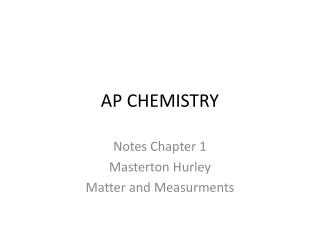DownloadDownload PresentationAP CHEMISTRY

# AP CHEMISTRY

Télécharger la présentation## AP CHEMISTRY

- - - - - - - - - - - - - - - - - - - - - - - - - - - E N D - - - - - - - - - - - - - - - - - - - - - - - - - - -
##### Presentation Transcript

1. AP CHEMISTRY Notes Chapter 1 Masterton Hurley Matter and Measurments

2. Types of Substances • Separating Mixtures • Filtration: Mixture passes through a barrier with fine pores leaving the solid on the barrier. • Distillation: Liquid vaporizes leaving a residue of the solid behind. • Chromatography: Uses solubility and adsorption to separate components.

3. Measured quantities • Length SI unit m meter Tiny distances are measured in nanometers, 10-9 m • Volume SI unit L Liter 1L = 103mL = 103 cm3 And 1 mL = 1 cm3 • Mass SI unit g gram 1Kg = 103g • Temperature SI unit ˚C celsius Conveting to Kelvin, K, = ˚C + 273.15

4. Experimental ErrorSignificant Figures Accuracy of tools used determine the significant figures. decigram balance 26.3 g the decigram balance gives you 3 s.f. analytical balance 26.582g the analytical balance gives you 5 s.f. An analytical balance measures accurately to the one thousandth of a gram.

5. Significant figures Multiplying and dividing= the answer has the fewest significant figures. 10.2 x 12.5423 = 127.93146 3sf 6sf reported answer should have 3sf 128 Adding and subtracting= the answer has the least significant place value. 16 - 1.895 = 14.105 Ones thousandths reported answer should be significant to the ones place 14

6. Conversion factors • Changing from one unit to another • Multiply your starting value by a conversion factor • Conversion factors have a value of 1 1.376 USD \$ = 1.000 Euro € Convert 5.51 € to \$ Choose a factor that cancels the initial unit.

7. Convert 89.6 miles/hour to a value in meters per second. 1 mile = 1.609 km 1 Km = 1000 m 1 hr = 3600s Show all work, and cancel units.

8. Properties of substances • Intensive property • Can be used to identify a substance, the value is independent of the amount. • Ex. Density, Boiling Point. • Extensive property • Is dependent upon the amount of substance. • Ex. Mass, volume.

9. Identifying substances • Chemical properties • Observed when a substance takes part in a chemical reaction. • Physical properties • Observed without changing the chemical identity of the substance. • Ex. Melting point, boiling point, density, hardness

10. Properties of Substances • Density d=m/v A flask containing 5.00mL of octane has a mass of 25.598g. The empty flask has a mass of 22.138g. What is the density of the octane? What would be the volume of 10.00g of octane?

11. Properties of Substances • Solubility • An amount of solute that is dissolved in a solvent. • Often expressed as g of solute/ 100 g solvent • Ex. Solubility of Sugar @20˚C = 210g sugar/100g water • A solution of 100g of water with 210g of sugar = saturated • A solution of 100g of water with <210g of sugar = unsaturated • A solution of 100g of water with >210g of sugar = supersaturated

12. Color and Absorption Spectrum • Visible light: 400nm – 700nm VIBGYOR <400nm >700nm ultra violet infra red

13. Solving for solubility • How much water is required to dissolve 52g of sugar (@20˚C) ? • A 20˚C solution contains 25g of sugar and 125 g of water. Is it saturated, unsaturated, or supersaturated?